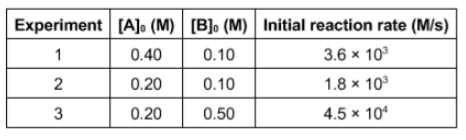# Problem: Kinetic data for the following reaction were determined experimentally: A + B → 2 CWhat is the rate law for the reaction?A. rate = k•[A]1•[B]2B. rate = k•[A]1/2•[B]5C. rate = k•[A]2•[B]1D. rate = k•[A]1•[B]1/5E. rate = k•[A]1/2•[B]2

###### FREE Expert Solution

We’re being asked to determine the rate law for the reaction based on the reaction and data given.

Recall that the rate law only focuses on the reactant concentrations and has a general form of:

k = rate constant
A & B = reactants
x & y = reactant orders

We’re going to calculate the rate law and the rate constant using the following steps:

79% (439 ratings)###### Problem Details

Kinetic data for the following reaction were determined experimentally:

A + B → 2 CWhat is the rate law for the reaction?

A. rate = k•[A]1•[B]2

B. rate = k•[A]1/2•[B]5

C. rate = k•[A]2•[B]1

D. rate = k•[A]1•[B]1/5

E. rate = k•[A]1/2•[B]2